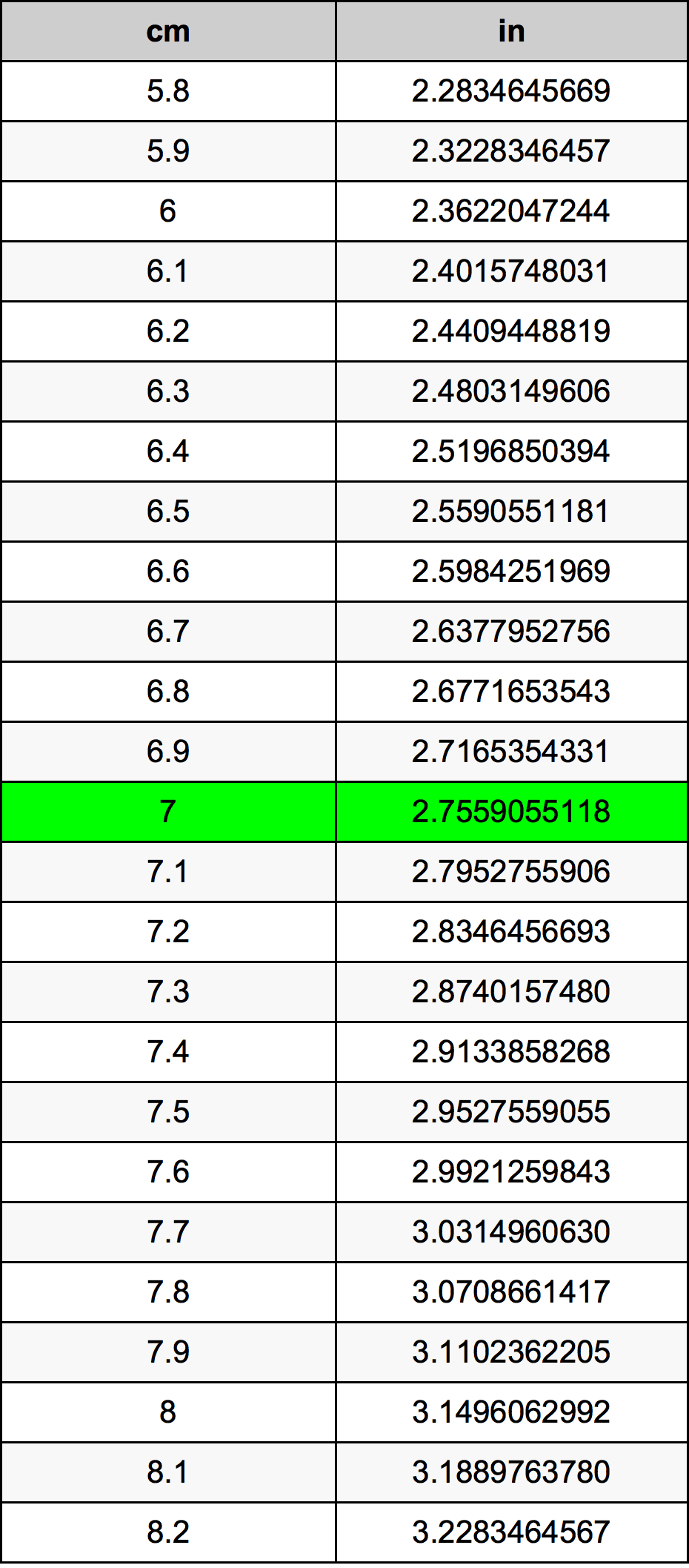Cm To Inches

# 7 cm to in7 Centimeters to Inches

cm
=
in

## How to convert 7 centimeters to inches?

 7 cm * 0.3937007874 in = 2.7559055118 in 1 cm
A common question is How many centimeter in 7 inch? And the answer is 17.78 cm in 7 in. Likewise the question how many inch in 7 centimeter has the answer of 2.7559055118 in in 7 cm.

## How much are 7 centimeters in inches?

7 centimeters equal 2.7559055118 inches (7cm = 2.7559055118in). Converting 7 cm to in is easy. Simply use our calculator above, or apply the formula to change the length 7 cm to in.

## Convert 7 cm to common lengths

UnitLength
Nanometer70000000.0 nm
Micrometer70000.0 µm
Millimeter70.0 mm
Centimeter7.0 cm
Inch2.7559055118 in
Foot0.2296587927 ft
Yard0.0765529309 yd
Meter0.07 m
Kilometer7e-05 km
Mile4.3496e-05 mi
Nautical mile3.7797e-05 nmi

## What is 7 centimeters in in?

To convert 7 cm to in multiply the length in centimeters by 0.3937007874. The 7 cm in in formula is [in] = 7 * 0.3937007874. Thus, for 7 centimeters in inch we get 2.7559055118 in.

## 7 Centimeter Conversion Table## Alternative spelling

7 Centimeter to Inch, 7 Centimeter in Inch, 7 Centimeters to in, 7 Centimeters in in, 7 Centimeter to Inches, 7 Centimeter in Inches, 7 cm to Inch, 7 cm in Inch, 7 Centimeter to in, 7 Centimeter in in, 7 Centimeters to Inch, 7 Centimeters in Inch, 7 cm to Inches, 7 cm in Inches